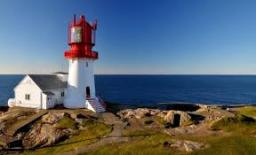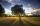# Lighthouse

The man, 180 cm tall, walks along the seafront directly to the lighthouse. The male shadow caused by the beacon light is initially 5.4 meters long. When the man approaches the lighthouse by 90 meters, its shadow shorter by 3 meters. How tall is the lighthouse and how far is the man away from it?

Correct result:

a =  54 m
b =  162 m

#### Solution:

a = 1.8 / 5.4 b
a = 1.8 / (5.4-3)•(b-90)

a = 1.8 / 5.4•b
a = 1.8 / (5.4-3)•(b-90)

a-0.333333b = 0
a-0.75b = -67.5

a = 54
b = 162

Our linear equations calculator calculates it.We would be pleased if you find an error in the word problem, spelling mistakes, or inaccuracies and send it to us. Thank you!Tips to related online calculators
Do you have a linear equation or system of equations and looking for its solution? Or do you have quadratic equation?

#### You need to know the following knowledge to solve this word math problem:

We encourage you to watch this tutorial video on this math problem:

## Next similar math problems:Tree perpendicular to the horizontal surface has a shadow 8.32 meters long. At the same time meter rod perpendicular to the horizontal surface has shadow 64 cm long. How tall is tree?Miro stands under a tree and watching its shadow and shadow of the tree. Miro is 180 cm tall and its shade is 1.5 m long. The shadow of the tree is three times as long as Miro's shadow. How tall is the tree in meters?Nine meters height poplar tree has a shadow 16.2 meters long. How long shadow have at the same time Joe if he is 1,4m tall?
• Right angled triangleHypotenuse of a right triangle is 17 cm long. When we decrease length of legs by 3 cm then decrease its hypotenuse by 4 cm. Determine the size of legs.
• Sides of right angled triangleOne leg is 1 m shorter than the hypotenuse, and the second leg is 2 m shorter than the hypotenuse. Find the lengths of all sides of the right-angled triangle.
• An observerAn observer standing west of the tower sees its top at an altitude angle of 45 degrees. After moving 50 meters to the south, he sees its top at an altitude angle of 30 degrees. How tall is the tower?
• Conical bottleWhen a conical bottle rests on its flat base, the water in the bottle is 8 cm from it vertex. When the same conical bottle is turned upside down, the water level is 2 cm from its base. What is the height of the bottle?
• Two chordsCalculate the length of chord AB and perpendicular chord BC to circle if AB is 4 cm from the center of the circle and BC 8 cm from the center of the circle.How long is a ladder that touches on a wall 4 meters high and its lower part is 3 meters away from the wall?
• Isosceles trapezoidCalculate the content of an isosceles trapezoid whose bases are at ratio 5:3, the arm is 6cm long and it is 4cm high.
• LighthouseMarcel (point J) lies in the grass and sees the top of the tent (point T) and behind it the top of the lighthouse (P). | TT '| = 1.2m, | PP '| = 36m, | JT '| = 5m. Marcel lies 15 meters away from the sea (M). Calculate the lighthouse distance from the sea
• Vertical rodThe vertical one meter long rod casts a shadow 150 cm long. Calculate the height of a column whose shadow is 36 m long at the same time.
• Similar trianglesTriangle A'B'C 'is similar to triangle ABC, whose sides are 5 cm, 8 cm, and 7 cm long. What is the length of the sides of the triangle A'B'C ' if its circumference is 80 cm?
• TrapezoidHow long are the trapezoid bases with area 24 cm2 and height 3 cm. One base is 3 times longer than the shorter.
• Euclid theoremsCalculate the sides of a right triangle if leg a = 6 cm and a section of the hypotenuse, which is located adjacent the second leg b is 5cm.
• Area of iso-trapFind the area of an isosceles trapezoid if the lengths of its bases are 16 cm and 30 cm, and the diagonals are perpendicular to each other.
• GarageThere are two laths in the garage opposite one another: one 2 meters long and the second 3 meters long. They fall against each other and stay against the opposite walls of the garage and both laths cross 70 cm above the garage floor. How wide is the garag